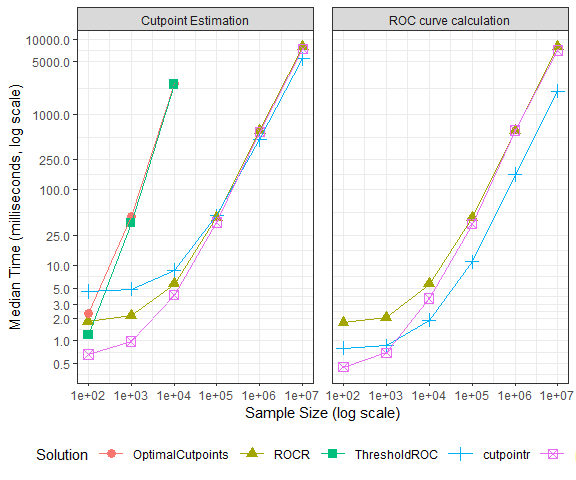# cutpointr benchmarks

#### 2022-04-13

To offer a comparison to established solutions, cutpointr will be benchmarked against optimal.cutpoints from the OptimalCutpoints package, ThresholdROC and custom functions based on the ROCR and pROC packages. By generating data of different sizes the benchmarks will offer a comparison of the scalability of the different solutions.

Using prediction and performance from the ROCR package and roc from the pROC package, we can write functions for computing the cutpoint that maximizes the sum of sensitivity and specificity. pROC has a built-in function to optimize a few metrics:

# Return cutpoint that maximizes the sum of sensitivity and specificiy
# ROCR package
rocr_sensspec <- function(x, class) {
pred <- ROCR::prediction(x, class)
perf <- ROCR::performance(pred, "sens", "spec")
sens <- slot(perf, "y.values")[]
spec <- slot(perf, "x.values")[]
cut <- slot(perf, "alpha.values")[]
cut[which.max(sens + spec)]
}

# pROC package
proc_sensspec <- function(x, class) {
r <- pROC::roc(class, x, algorithm = 2, levels = c(0, 1), direction = "<")
pROC::coords(r, "best", ret="threshold", transpose = FALSE)
}

The benchmarking will be carried out using the microbenchmark package and randomly generated data. The values of the x predictor variable are drawn from a normal distribution which leads to a lot more unique values than were encountered before in the suicide data. Accordingly, the search for an optimal cutpoint is much more demanding, if all possible cutpoints are evaluated.

Benchmarks are run for sample sizes of 100, 1000, 1e4, 1e5, 1e6, and 1e7. For low sample sizes cutpointr is slower than the other solutions. While this should be of low practical importance, cutpointr scales more favorably with increasing sample size. The speed disadvantage in small samples that leads to the lower limit of around 25ms is mainly due to the nesting of the original data and the results that makes the compact output of cutpointr possible. This observation is emphasized by the fact that cutpointr::roc is quite fast also in small samples. For sample sizes > 1e5 cutpointr is a little faster than the function based on ROCR and pROC. Both of these solutions are generally faster than OptimalCutpoints and ThresholdROC with the exception of small samples. OptimalCutpoints and ThresholdROC had to be excluded from benchmarks with more than 1e4 observations due to high memory requirements and/or excessive run times, rendering the use of these packages in larger samples impractical.

# ROCR package
rocr_roc <- function(x, class) {
pred <- ROCR::prediction(x, class)
perf <- ROCR::performance(pred, "sens", "spec")
return(NULL)
}

# pROC package
proc_roc <- function(x, class) {
r <- pROC::roc(class, x, algorithm = 2, levels = c(0, 1), direction = "<")
return(NULL)
}n task OptimalCutpoints ROCR ThresholdROC cutpointr pROC
1e+02 Cutpoint Estimation 2.288702 1.812802 1.194301 4.5018015 0.662101
1e+03 Cutpoint Estimation 45.056801 2.176401 36.239852 4.8394010 0.981001
1e+04 Cutpoint Estimation 2538.612001 5.667101 2503.801251 8.5662515 4.031701
1e+05 Cutpoint Estimation NA 43.118751 NA 45.3845010 37.150151
1e+06 Cutpoint Estimation NA 607.023851 NA 465.0032010 583.095000
1e+07 Cutpoint Estimation NA 7850.258700 NA 5467.3328010 7339.356101
1e+02 ROC curve calculation NA 1.732651 NA 0.7973505 0.447701
1e+03 ROC curve calculation NA 2.035852 NA 0.8593010 0.694802
1e+04 ROC curve calculation NA 5.662151 NA 1.8781510 3.658050
1e+05 ROC curve calculation NA 42.820852 NA 11.0992510 35.329301
1e+06 ROC curve calculation NA 612.471901 NA 159.8100505 610.433700
1e+07 ROC curve calculation NA 7806.385452 NA 2032.6935510 7081.897251